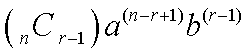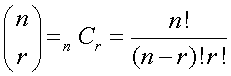Chapter 11: Sequences and Series

 Arithmetic Note: SequenceArithmetic Sequence Series (Find the sum)When you know the first and last term.When you know the first term and the common difference. Geometric Note: Sequence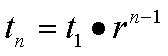Geometric Sequence Series (Find the sum)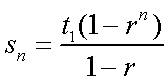A finite Geometric Series (a limited number of terms, or Partial Sum)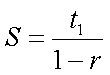An infinite Geometric Series, if our infinite series is convergent (| r | < 1)

# Binomial Expansion

To find all terms in a Binomial Expansion, use: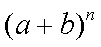=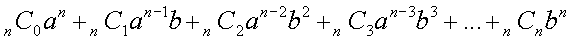To find the rth term of a binomial expansion raised to the nth power, use the following formula: